# 多线程---volatile关键字

• 内存结构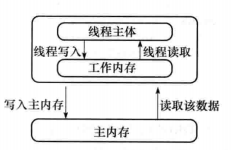有这个结构图，就能很清晰的知道为毛会造成数据的不同步了。每个线程都会有各自的线程栈，执行运算的时候，是从公共堆栈读取数据到线程堆栈中，线程操作的是线程堆栈的数据，结束后，再从线程堆栈回刷到公共堆栈，所以这种肯定会引起数据的不同步。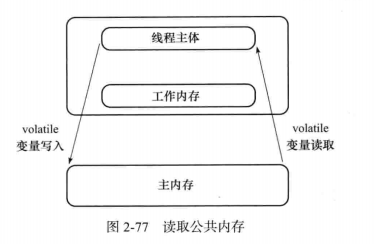public class MyThread extends Thread {
private boolean isRun= true;

@Override
public void run() {
System.out.println("run start");
while(isRun){

}
System.out.println("run end");
}

public void setRun(boolean isRun) {
this.isRun = isRun;
}

}

public static void main(String[] args) throws Exception {
r.start();
r.setRun(false);

}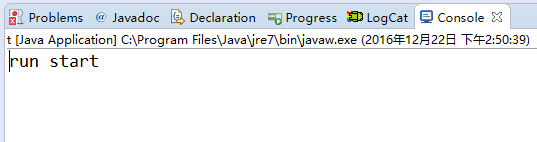public class MyThread extends Thread {
volatile    private boolean isRun= true;

@Override
public void run() {
System.out.println("run start");
while(isRun){

}
System.out.println("run end");
}

public void setRun(boolean isRun) {
this.isRun = isRun;
}

}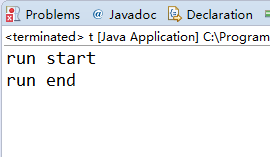• 非原子性
来个demo验证：
public class MyThread extends Thread {
volatile public static int count;
@Override
public void run() {
}

for(int i=0;i<100;i++){
count++;
}
System.out.println("count="+count);
}
}

public static void main(String[] args) throws Exception {
for(int i=0;i<rs.length;i++){
}

r.start();
}

}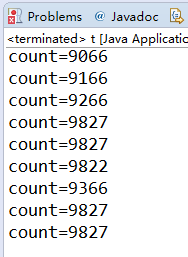synchronized private static void add() {
for(int i=0;i<100;i++){
count++;
}
System.out.println("count="+count);
}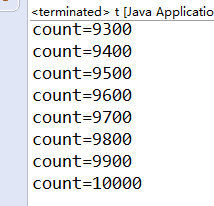1）从内存中取i的值
2)计算i的值
3）将i的值写到内存中。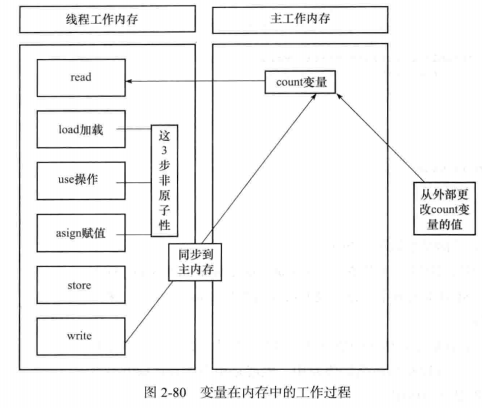• 原子类进行操作
原子操作是不能分割的整体，没有其他线程能够中断或检查正在原子操作中的变量，一个原子类型（Atomicxxx）就是一个原子操作可用的类型，他可以在没有锁的情况下做到线程安全。
public class MyThread extends Thread {
private AtomicInteger atomic = new AtomicInteger(0);

@Override
public void run() {
}

for(int i=0;i<100;i++){
System.out.println("count="+atomic.incrementAndGet());
}

}
}

public static void main(String[] args) throws Exception {
for(int i=0;i<rs.length;i++){
}

m.start();
}

}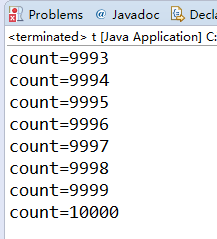• 原子类也并不完全安全
public class MyThread extends Thread {
public static AtomicInteger atomic = new AtomicInteger(0);

@Override
public void run() {
}

}

}

public static void main(String[] args) throws Exception {
for(int i=0;i<rs.length;i++){
}

m.start();
}

}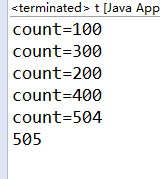public class MyThread extends Thread {
public static AtomicInteger atomic = new AtomicInteger(0);

@Override
public void run() {
}

}

}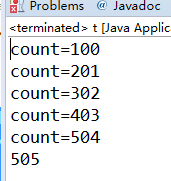©️2019 CSDN 皮肤主题: 大白 设计师: CSDN官方博客